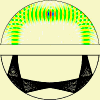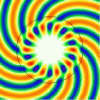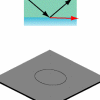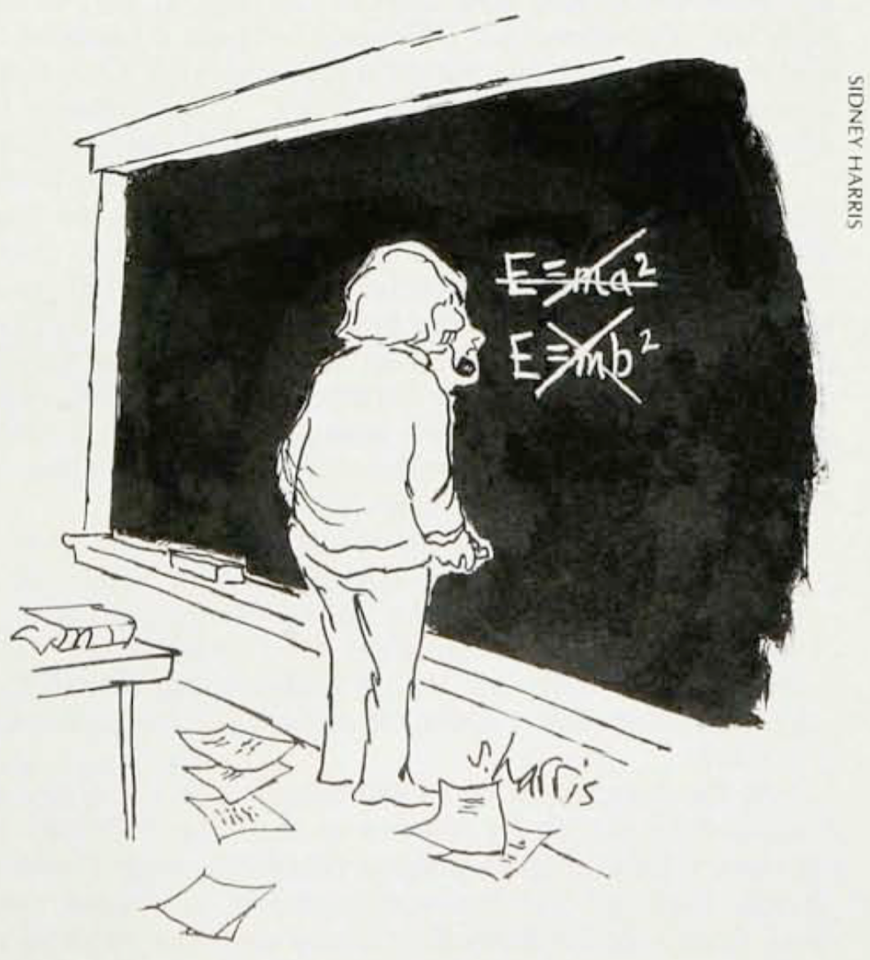• SearchHide Search
• Home• Vitae• News• Papers• Microlasers• Research• Teaching• Address# Maxwell's Equations as Mechanical Law

## Do the foundations of electromagnetism require a variational principle?

Maxwell's equations are a concise statement of the fundamental laws of electrodynamics — the field of physics and engineering dedicated to the interaction between material objects via electromagnetic forces.Albert Einstein's 1905 paper On the Electrodynamics of Moving Bodies can be regarded as the founding document of the special theory of relativity. This is the theory that superseded Newtonian mechanics by replacing the Galilean principle of relativity with a bold new idea: that time is not absolute.

Einstein was led to special relativity by the laws of electrodynamics.

Even the teaching of introductory electromagnetism can't really do without Einstein, if one looks carefully. It starts with the Lorentz force law, relating the force on a charged object to its velocity. Any student looking at this law will be perplexed by it, if they know only the laws of Newtonian and Galilean relativity.

The Lorentz force law declares the electromagnetic force to be linearly related to an object's velocity. But force is independent of the (inertial) reference frame of the observer, whereas the velocity of an object is relative. Einstein's relativistic mechanics fixes this problem by redefining the concept of inertial frames.

But it is now known that the axioms of relativistic mechanics can be written in a way that makes no use whatsoever of any facts from electromagnetism at the outset. In particular, there needs to be no reference to the speed of light, only to the fact that some maximum attainable speed exists for all mechanical objects. With this knowledge, one can ask whether electromagnetism as a whole is a logical consequence of relativistic mechanics.

In the paper "Maxwell's Equations as Mechanical Law" I follow this logical framework and show how electrodynamics, and with it the speed of light as the limiting speed of mechanics, can be derived from the version of relativistic mechanics just described. The guiding principle in my derivation is that we should not be required to make any large leaps of faith that go beyond familiar laws of mechanics. The largest leap of faith would of course be to simply postulate that Maxwell's equations are true, period.

An almost equivalent leap of faith is to postulate a certain variational principle with a certain Lagrangian density to be true, which after standard manipulations will produce the equations we want it to produce. The variational principle is an elegant language in which to formulate laws of nature that makes it especially easy to see that these laws are invariant under various "changes of viewpoint". But if you simply reformulated a law of nature in a different language, that doesn't mean you have derived it.

One important insight in my derivation of Maxwell's equations is that the process of arriving at the laws of electrodynamics from the postulates of relativistic mechanics has built into it the Newtonian force concept, according to which objects by definition do not exert forces on themselves. This leads to the distinction between the electromagnetic field created by a distribution of charges, versus the fields external to that distribution. The latter can be varied while the former stay the same, and imposing this requirement is equivalent to Maxwell's equations. The biggest leap of faith we have to take is to accept the mechanically motivated hypothesis that the law of energy- and momentum conservation should hold during an electromagnetic interaction.